Problem

# Sketch vo for each network of Fig. 2.182 for the input shown.FIG. 2.182

Sketch vo for each network of Fig. 2.182 for the input shown.

FIG. 2.182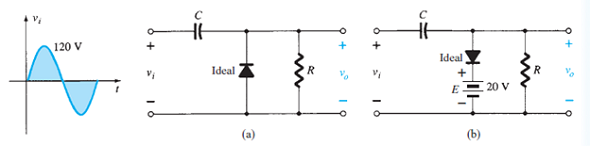#### Step-by-Step Solution

Solution 1

Refer to Figure 2.182 (a) in the text book.

(a)

Diode is connected in reverse bias, so first consider the negative values of input voltage,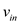.

Draw the following circuit diagram when the diode is short circuited.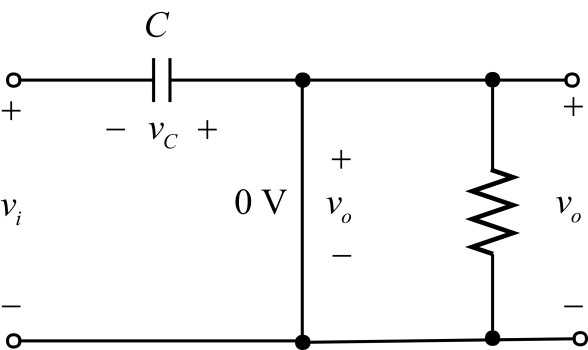Figure 1

Apply Kirchhoff’s voltage law to calculate the capacitor voltage.For positive values of input voltage,diode acts as open circuit.

Draw the following circuit diagram for positive values of input voltage,.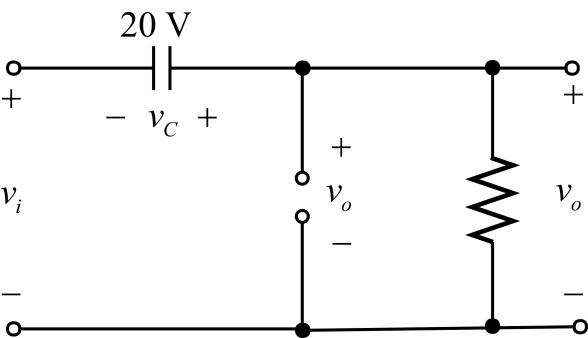Figure 2

Apply Kirchhoff's voltage law to calculate the output voltage.

$$v_{i}+v_{C}-v_{o}=0$$

$$v_{o}=v_{i}+v_{C}$$

$$v_{o}=v_{i}+120$$

The input voltage ranges from $$-120 \mathrm{~V}$$ to $$+120 \mathrm{~V}$$.

Calculate the output voltage.

$$v_{o}=(-120+120)$$ to $$(+120+120)$$

$$=0 \mathrm{~V}$$ to $$240 \mathrm{~V}$$

Draw the following output waveform: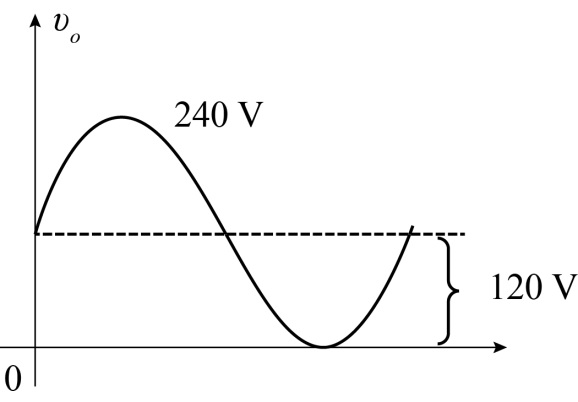Figure 3

(b)

Refer to Figure 2.182 (b) in the text book.

Diode is connected in forward bias, so first consider the positive values of input voltage,.

Draw the following circuit diagram when the diode is short circuited.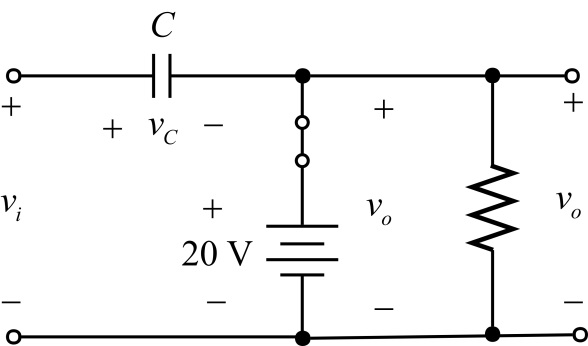Figure 4

Apply Kirchhoff’s voltage law to calculate the capacitor voltage.For positive values of input voltage,diode acts as open circuit.

Draw the following circuit diagram for positive values of input voltage,.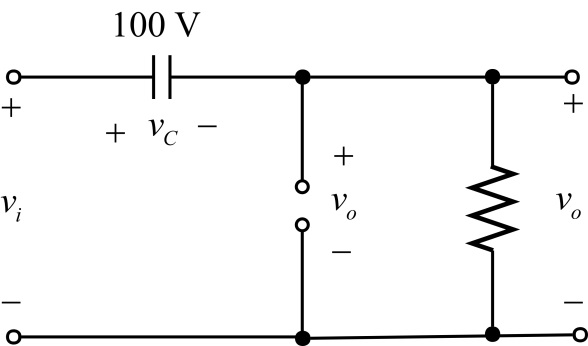Figure 5

Apply Kirchhoff's voltage law to calculate the output voltage.

$$-v_{i}+v_{c}+v_{o}=0$$

$$v_{o}=v_{1}-v_{C}$$

$$v_{o}=v_{i}-100$$

The input voltage ranges from $$-120 \mathrm{~V}$$ to $$+120 \mathrm{~V}$$.

Calculate the output voltage.

\begin{aligned} v_{o} &=(-120-100) \text { to }(120-100) \\ &=-220 \mathrm{~V} \text { to } 20 \mathrm{~V} \end{aligned}

Draw the following output waveform: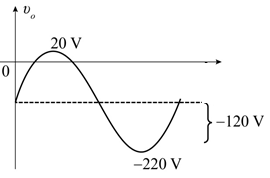Figure 6